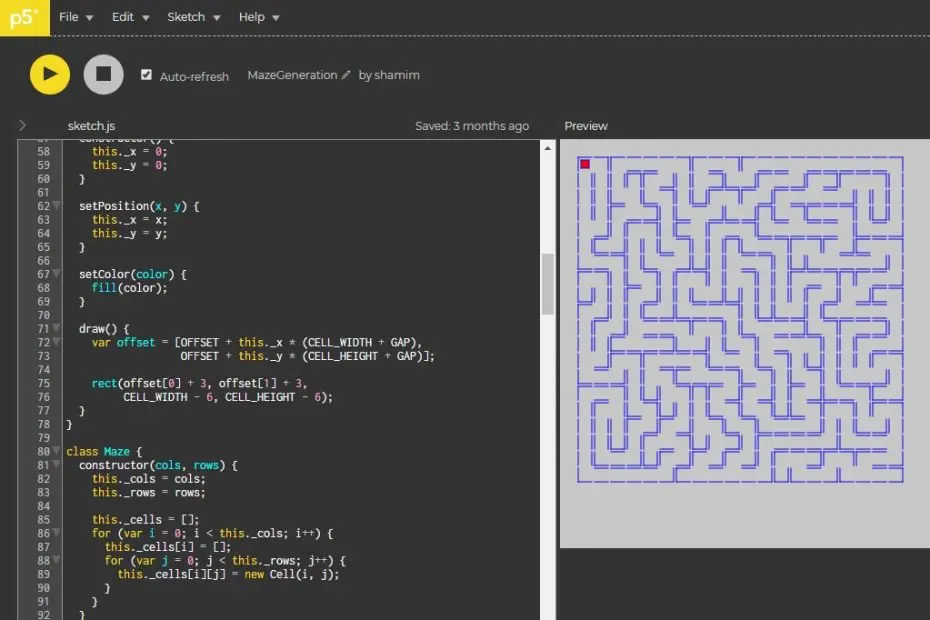# Depth-First## How to Generate Mazes Using Depth-First Algorithm

In this tutorial, we will learn how to generate mazes using a depth-first algorithm. To do this firstly, I will define the problem, then I will explain the algorithm and finally demonstrate the implementation in… Read More »How to Generate Mazes Using Depth-First Algorithm## Solving 8 puzzle problem using A* star search in C++

In this tutorial, we will solve the 8-puzzle problem using A* star search in C++. This tutorial is Part 2 of the tutorial series on Solving 8 puzzle problem using A* search. Contact me Find… Read More »Solving 8 puzzle problem using A* star search in C++